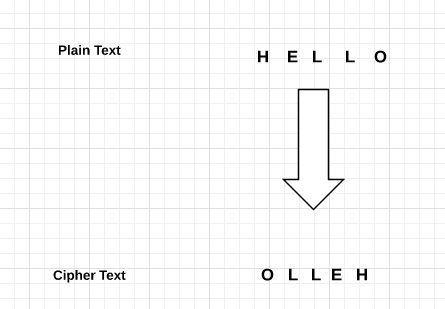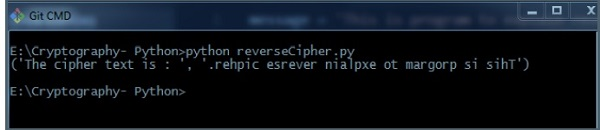# Cryptography with Python – Reverse Cipher

The previous chapter gave you an overview of installation of Python on your local computer. In this chapter you will learn in detail about reverse cipher and its coding.

## Algorithm of Reverse Cipher

The algorithm of reverse cipher holds the following features −

• Reverse Cipher uses a pattern of reversing the string of plain text to convert as cipher text.

• The process of encryption and decryption is same.

• To decrypt cipher text, the user simply needs to reverse the cipher text to get the plain text.

## Drawback

The major drawback of reverse cipher is that it is very weak. A hacker can easily break the cipher text to get the original message. Hence, reverse cipher is not considered as good option to maintain secure communication channel,.### Example

Consider an example where the statement This is program to explain reverse cipher is to be implemented with reverse cipher algorithm. The following python code uses the algorithm to obtain the output.

```message = 'This is program to explain reverse cipher.'
translated = '' #cipher text is stored in this variable
i = len(message) - 1

while i >= 0:
translated = translated + message[i]
i = i - 1
print(“The cipher text is : “, translated)```

### Output

You can see the reversed text, that is the output as shown in the following image −### Explanation

• Plain text is stored in the variable message and the translated variable is used to store the cipher text created.

• The length of plain text is calculated using for loop and with help of index number. The characters are stored in cipher text variable translated which is printed in the last line.